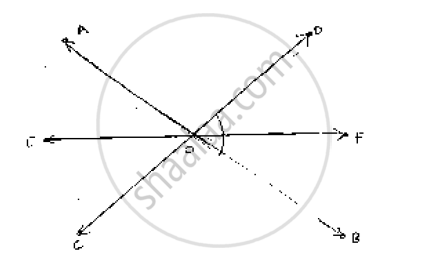# Ab, Cd and Ef Are Three Concurrent Lines Passing Through the Point O Such that of Bisects ∠Bod. If ∠Bof = 35°, Find ∠Boc and ∠Aod. - Mathematics

AB, CD and EF are three concurrent lines passing through the point O such that OF bisects
∠BOD. If ∠BOF = 35°, find ∠BOC and ∠AOD.

#### SolutionGiven

OF bisects  ∠BOD

OF bisects  ∠BOD

∠BOF = 35°

∠BOC = ?

∠AOD = ?

∴∠BOD = 2 ∠BOF = 70°                    [ ∵ of bisects ∠BOD]

∠BOD = ∠AOC = 70°              [ ∠BODand ∠AOCare vertically opposite angles]

Now,

∠BOC + ∠AOC = 180°

⇒ ∠BOC + 70° = 180°

⇒∠BOC = 110°

∴ ∠AOD = ∠BOC = 110°               [Vertically opposite angles]

Concept: Pairs of Angles
Is there an error in this question or solution?

#### APPEARS IN

RD Sharma Mathematics for Class 9
Chapter 10 Lines and Angles
Exercise 10.3 | Q 8 | Page 23